﻿ Why Heat Transfer Coefficients Are Unnecessary and Undesirable, and How Heat Transfer Problems Are Solved without ThemPublications are Open
Access in this journal
Article Versions
Export Article
• Normal Style
• MLA Style
• APA Style
• Chicago Style
Research Article
Open Access Peer-reviewed

### Why Heat Transfer Coefficients Are Unnecessary and Undesirable, and How Heat Transfer Problems Are Solved without Them

American Journal of Mechanical Engineering. 2020, 8(3), 106-110. DOI: 10.12691/ajme-8-3-2
Received August 09, 2020; Revised September 08, 2020; Accepted September 17, 2020

### Abstract

For 200 years, convective heat flux q has been calculated by multiplying heat transfer coefficient h times boundary layer temperature difference ΔT. Since h times ΔT equals q, h must be a symbol for (q/ΔT) because (q/ΔT) times ΔT equals q. h (ie q/ΔT) is generally calculated from correlations derived from experiments in which q data and ΔT data are used to obtain (q/ΔT){ΔT} correlations-ie h{ΔT} correlations. (It is not possible to obtain h data because h is not a parameter. h is the ratio of two parameters). Heat transfer coefficients are unnecessary and undesirable. It is self-evident that any problem that can be solved using q, q/ΔT (ie h), and ΔT can also be solved using only q and ΔT. Therefore h (ie q/ΔT) is unnecessary. h (ie q/ΔT) is undesirable because, when q is a nonlinear function of ΔT (as in free convection, condensation, and boiling), h (ie q/ΔT) is an extraneous variable, and it greatly complicates problem solutions. When h has been abandoned, convective heat flux is determined from q{ΔT} correlations that result from q data and ΔT data, or from the transformation of h{ΔT} correlations. (Transformation from h{ΔT} correlations to q{ΔT} correlations requires that h be replaced by q/ΔT, and that q and ΔT be separated.). The text includes example problems that validate the conclusion that h (ie q/ΔT) is unnecessary and undesirable, and demonstrate that the solution of nonlinear problems is much simpler if h is abandoned.

### 1. Introduction

For 200 years, convection heat flux q has been determined by multiplying heat transfer coefficient h times boundary layer temperature difference ΔT. Critical appraisal of this methodology indicates that it greatly complicates the solution of problems that concern nonlinear thermal behavior (such as natural convection, condensation, and boiling), and it should be replaced by methodology that allows the simplest possible solution of such problems.

### 2. What Exactly is h?

Equations (1) and (2) are identical. Both equations state that h is a symbol for q/ΔT.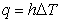(1)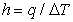(2)

Nomenclatures in every heat transfer text should state “h is a symbol for q/ΔT, and is named “heat transfer coefficient”. (Nomenclatures in most heat transfer texts state only that h is named “heat transfer coefficient”.)

### 3. The Genesis of h and Eq. (1).

American heat transfer texts generally refer to Eq. (1) as “Newton’s law of cooling”, and credit Newton 1 with h and Eq. (1). However, 2 and 3 state that Fourier 4 should be credited with h and Eq. (1). Equation (1) cannot be “Newton’s law of cooling” because cooling is a transient phenomenon, and Eq. (1) is a steady-state equation.

Fourier performed experiments on steady-state forced convection heat transfer to ambient air. He conceived h and Eq. (1) to correlate his data. In Fourier’s view, Eq. (1):

Ÿ States that, if heat transfer is by steady-state forced convection to ambient air, q is always proportional to ΔT.

Ÿ States that, if heat transfer is by steady-state forced convection to ambient air, h is always a constant of proportionality.

Ÿ Defines h to be a symbol for q/ΔT.

Ÿ Is the law of steady-state forced convection heat transfer to ambient air. It is a law because it is always obeyed.

### 4. The Modern Meaning of Eq. (1) and h.

Sometime near the beginning of the twentieth century, it was decided to apply Eq. (1) to nonlinear forms of convection heat transfer such as natural convection, condensation, and boiling. This decision had the following effects on the meaning of Eq. (1) and h:

Ÿ Equation (1) no longer meant that the relationship between q is always proportional to ΔT. It thereafter meant that the relationship between q and ΔT may be proportional or nonlinear.

Ÿ Equation (1) no longer meant that h is always a proportionality constant. It thereafter meant that h may be a proportionality constant or a variable dependent on ΔT.

Ÿ Equation (1) continued to define h to be a symbol for q/ΔT.

### 5. Why the Modern Eq. (1) is neither an Equation nor a Law

It is self-evident that a proportional equation cannot describe nonlinear behavior. Therefore Eq. (1) ceased to be an equation when it began to apply to nonlinear behavior. Because Eq. (1) ceased to be an equation, it ceased to be a law. There has in fact been no law of convective heat transfer since Eq. (1) ceased to be a law.

### 6. Why h (ie q/ΔT) is Unnecessary

h (ie q/ΔT) is unnecessary because, if a problem can be solved using q, q/ΔT (ie h), and ΔT, it can also be solved using only q and ΔT.

### 7. Why h (ie q/ΔT) is Undesirable

If the relationship between q and ΔT is proportional (as in forced convection heat transfer to one phase fluids), h (ie q/ΔT) is a constant, and Eq. (1) contains the two variables q and ΔT. But if the relationship is nonlinear (as in free convection, condensation, and boiling), h (ie q/ΔT) is a variable, and Eq. (1) contains the three variables q, h (ie q/ΔT), and ΔT.

h (ie q/ΔT) is undesirable because, if the relationship between q and ΔT is nonlinear, h (ie q/ΔT) is an extraneous variable that greatly complicates solutions.

### 8. How Convective Heat Flux Is Calculated after h Has Been Abandoned

After h has been abandoned, q is calculated from q{ΔT} correlations based on q data and ΔT data, or from q{ΔT} correlations obtained by the transformation of h{ΔT} correlations. (The transformation requires that h be replaced by q/ΔT, and that q and ΔT be separated.)

### 9. How to Eliminate h (ie q/ΔT) in Heat Transfer Texts

In order to eliminate h in heat transfer texts, substitute q/ΔT for h and for k/t in all equations that implicitly or explicitly include h, then separate q and ΔT. For example, Eq. (5) is used to analyze heat transfer between two fluids separated by a flat wall. (U is overall heat transfer coefficient q/ΔTtotal, twall is wall thickness, kwall is thermal conductivity of the wall.)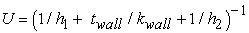(5)

To separate q and ΔT in Eq. (5):

Ÿ Substitute q/ΔTtotal for U. Substitute q/ΔT1 for h1 and q/ΔT2 for h2.

Ÿ Substitute q/ΔTwall for kwall/twall.

Ÿ Separate q and ΔT, resulting in Eq. (6).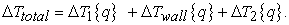(6)

Equations (5) and (6) are identical-they differ only in form. Therefore, any problem that can be solved using Eq. (5) and h can also be solved using Eq. (6) and not h.

Equation (7) is a heat transfer coefficient correlation often used in the analysis of forced convection heat transfer. (Nu is Nusselt number, D is diameter, Re is Reynolds number, Pr is Prandtl number.) To separate q and ΔT in Eq. (7), replace Nu with qD/ΔTk, then separate q and ΔT, resulting in Eq. (8a) or (8b).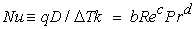(7)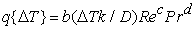(8a)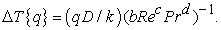(8b)

### 10. Without using h, Solve a Heat Transfer Problem in Which the Relationship between q and ΔT is Proportional (as in Forced Convection Heat Transfer)

10.1. Problem 1, Statement

Without using h, calculate the heat flux through a flat wall that separates Fluids 1 and 2.

10.2. Problem 1, Given

Parameter dimension units are specified in Nomenclature.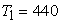(9)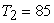(10)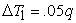(11)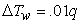(12)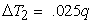(13)(14)
10.3. Problem 1, Analysis and Solution

Combine Eqs. (11 to (14), resulting in Eq. (15).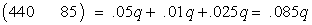(15)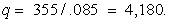(16)
10.4. Problem 1, Conclusions

Problem 1 is simple to solve whether h is or is not used in the solution because q/ΔT is a constant. Consequently the problem solution contains two thermal variables whether h is or is not used in the solution.

### 11. Without Using h, Solve a Heat Transfer Problem in which the Relationship between q and ΔT is Moderately Nonlinear (as in Free Convection Heat Transfer).

11.1. Problem 2, Statement

Calculate the heat flux through a flat wall that separates Fluids 1 and 2.

11.2. Problem 2, Given

Parameter dimension units are specified in Nomenclature.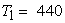(17)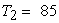(18)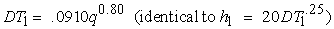(19)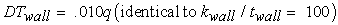(20)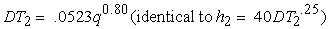(21)
11.3. Analysis and Solution, Problem 2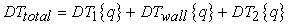(22)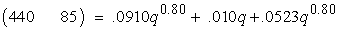(23)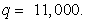(24)
11.4. Analysis of Problem 2 Using h Methodology

If h methodology is used, the analysis is based on Eq. (25), and results in Eq. (26).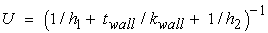(25)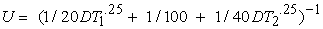(26)
11.5. Problem 2 Conclusions

Problem 2 demonstrates that moderately nonlinear problems are much more difficult to solve if h is used in the solution. Note that Eq. (23) has only one unknown variable, and can be solved by anyone in about a minute using Excel and trial-and-error methodology. Also note that Eq. (26) has three unknown variables (U, ΔT1, and ΔT2), and can be solved only by someone who knows a good deal about heat transfer and mathematics. Also, Eq. (26) takes much longer than a minute to solve, and has a much greater likelihood of error. In order to solve Eq. (26), it is necessary to:

Ÿ Find two more equations that apply to the problem.

Ÿ Solve the three equations simultaneously to determine q/ΔTtotal-ie to determine U.

Ÿ Multiply q/ΔTtotal times ΔTtotal to determine q.

### 12. Without Using h, Solve a Heat Transfer Problem in Which q is a Highly Nonlinear Function of ΔT

12.1. Statement, Problem 3

Given a vented pool boiler with a horizontal boiler plate, a boiling fluid above the boiler plate, and a heat source fluid below the boiler plate, determine the following without using h:

Ÿ The heat flux through the boiler plate at various heat source temperatures.

Ÿ The thermal stability of the boiler at various heat source temperatures.

Ÿ The effect of thermal instability on boiler behavior.

12.2. Given, Problem 3

Ÿ Parameter dimension units are specified in Nomenclature.

Ÿ The heat transfer behavior of the boiling interface is qualitatively described in Figure 1. The y axis is labeled qout because the chart concerns heat flux out of the boiling interface.

Ÿ The boiler heat source is Fluid 1 at temperature T1.

Ÿ The boiling fluid is Fluid 2 at temperature Tsat. Tsat is fixed because the boiler is vented.

Ÿ The behavior described in Figure 1 resembles the boiling behavior of liquid metals.

The heat transfer in Region 1 of Figure 1 is by natural convection from the boiler plate to the surface of Fluid 2, and by evaporation at the surface.

Ÿ Region 2 is a dashed line because there is no steady-state solution in Region 2. However, the boiler behaves as though the dashed line accurately describes functionality in Region 2.

Ÿ The heat transfer behavior of the boundary layer on the Fluid 1 surface of the boiler plate is described by Eq. (27). (b and c are arbitrary constants. Subscript bp refers to boiler plate.)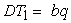(27)

Ÿ The heat transfer behavior of the boiler plate is described by Eq. (28).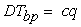(28)
12.3. How to Determine the Boiler Plate Heat Flux

Figure 1 describes the relationship between heat flux out of the boiling interface (qout) and the temperature of the boiling interface (Tbi). qin is the heat flux into the boiling interface. (440 - bqin - cqin) is the temperature of the boiling interface. On Figure 1, plot qin vs (440 - bqin - cqin). The boiler plate heat flux is the heat flux at intersections of qin and qout.

12.4. How to Appraise the Thermal Stability of the Boiler

The thermal stability of the boiler at an intersection of qin and qout is appraised by inspecting the intersection to determine whether a small perturbation would increase or decrease with time. If a small perturbation would decrease with time, the boiler is stable with regard to small perturbations. If a small perturbation would increase with time, the boiler is unstable. Symbolically, an intersection is thermally unstable if Inequality (29) is satisfied at the intersection.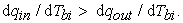(29)
• Figure 1. The thermal behavior of the boiling interface in Problem 3a
12.5. How to Determine the Boiler Behavior that Results from Thermal Instability

The boiler behavior that results from thermal instability is determined by inspecting intersections of qin and qout, and adjacent regions. If an intersection is thermally unstable, inspection of the chart will indicate either hysteresis or undamped oscillation in boiler plate temperature and heat flux.

12.5.1. The Thermal Stability and Boiler Behavior that Result if there is only One Intersection of qin and qout, and it is in Region 1, 3, 4, or 5 of Figure 1

If there is only one intersection of qin and qout, and it is in Region 1, 3, 4, or 5 of Figure 1, inspection of Figure 1 indicates that a small perturbation at the intersection would decrease with time. Therefore all intersections indicate thermal stability with regard to small perturbations. However, a boiler that is thermally stable with regard to small perturbations may be thermally unstable with regard to large perturbations.

12.5.2. Thermal Stability and Boiler Behavior if There is One Intersection of qin and qout, and It is in Region 2 of Figure 1

If there is only one intersection of qin and qout, and it is in Region 2 of Figure 1, inspection of the intersection and the adjacent regions indicates that small perturbations at the intersection increase with time, and result in undamped oscillations in heat flux and boiler plate temperature as operation jumps from the upper end of Region 1 to the upper part of Region 3, transitions to the lower end of Region 3, jumps down to the lower part of Region 1, transitions to the upper end of Region 1, jumps to the upper part of Region 3, etc.

12.5.3. Thermal Stability and Boiler Behavior if There are Three Intersections of qin and qout, and They Are in Regions 1, 2, and 3 of Figure 1

If there are three intersections of qin and qout, and they are in Regions 1, 2, and 3 of Figure 1, inspection of the intersections and adjacent regions indicates that:

Ÿ The boiler operates in a stable manner in either Region 1 or Region 3.

Ÿ There are step changes in qout as Tbi is monotonically increased from Region 1 to Region 3, and as Tbi is monotonically decreased from Region 3 to Region 1.

Ÿ There are no undamped oscillations because, if the boiler is initially at an intersection in Region 2, it will transition to an intersection in either Region 1 or Region 3 where it will operate in a stable manner.

12.6. Solution of Problem 3 Using h Methodology

It would be extremely difficult to solve Problem 3 using h methodology, as evidenced by the fact that an h methodology solution of a problem like Problem 3 is not found in American heat transfer texts or journals.

12.7. Conclusions Based on Problems 1 to 3

Ÿ Problem 1 demonstrates that proportional problems are easily solved whether h is or is not used in the solution.

Ÿ Problem 2 demonstrates that moderately nonlinear problems are much simpler to solve if h is not used in the solution.

Ÿ Problem 3 demonstrates that highly nonlinear problems are simple to solve if h methodology is not used in the solution. It would be extremely difficult to solve Problem 3 using h methodology, as evidenced by the fact that an h methodology solution of a problem like Problem 3 is not found in American heat transfer texts or journals.

### 13. Conclusions

Heat transfer coefficients should be abandoned because they are unnecessary and undesirable. Convection heat flux should be calculated from q{ΔT} correlations based on q data and ΔT data, or q{ΔT} correlations obtained by separating q and ΔT in h{ΔT} correlations. (The separation of q and ΔT requires replacing h with q/ΔT, then separating q and ΔT. Note that this step reverses the step in which q data are divided by and ΔT data in order to obtain (q/ΔT){ΔT} correlations.)

### References

  Newton, I., 1701, “A Scale of the Degrees of Heat”, Phil Trans Royal Soc (London), 22, p. 824. In article View Article  Adiutori, E. F., 1990, “Origins of the Heat Transfer Coefficient”, Mechanical Engineering, August, pp 46-50. In article  Bejan, A., 2013, Convection Heat Transfer, 4th edition, Wiley, p. 32. In article View Article  Fourier, J., (1822), The Analytical Theory of Heat, 1955 Dover edition of the 1878 English translation, The University Press, Article 36. In articleThis work is licensed under a Creative Commons Attribution 4.0 International License. To view a copy of this license, visit http://creativecommons.org/licenses/by/4.0/